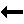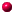What is this thing called Painlevé?contents Discrete and Difference Equations Much of what we know about the real world is modelled through continuous mathematical equations, i.e., differential equations, that become discrete equations on the computer. Consider the ordinary differential equation (ODE) $$y'=-y^2\qquad\qquad (0.1)$$ The derivative here can be approximated by using a Taylor expansion $$y(x+h)=y(x)+h\,y'(x)+O(h^2)\qquad (1.1)$$ for analytic functions $$y(x)$$, where $$h$$ is sufficiently small. So, letting $$\overline y = y(x+h)$$, we get $$y' = (\overline y - y)/h + O(h)$$ Excercise: Find an approximation for $$y''$$ by using a Taylor series expansion. Now the question arises: how do we replace the right side of Equation (0.1)?Back to ContentsNalini Joshi Last modified: 15 October 2013 by N.Joshi